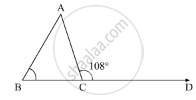Advertisement Remove all ads

# An Exterior Angle of a Triangle is 108° and Its Interior Opposite Angles Are in the Ratio 4 : 5. the Angles of the Triangle Are - Mathematics

MCQ

An exterior angle of a triangle is 108° and its interior opposite angles are in the ratio 4 : 5. The angles of the triangle are

#### Options

• 48°, 60°, 72°

•  50°, 60°, 70°

• 52°, 56°, 72°

• 42°, 60°, 76°

Advertisement Remove all ads

#### Solution

In the given ΔABC, an exterior angle  ∠ADC = 108° and its interior opposite angles are in the ratio 4:5.Let us take,

∠A = 4x

∠B = 5x

Now using the property, “exterior angle of a triangle is equal to the sum of two opposite interior angles”

We get,

∠A + ∠B = 108°

4x + 5x = 108°

9x = 108°

x = (108°)/ 9

x  = 12

Thus,

∠A = 4x = 4(12°) = 48°

∠B = 5x = 5(12°) = 60°

Also, using angle sum property in ΔABC

∠A + ∠B + ∠C = 180°

48° + 60° + ∠C = 180°

108° + ∠C = 180°

∠C = 180° - 108°

∠C = 72°

Thus,

∠A = 48°

∠B = 60°

∠C = 72°

Is there an error in this question or solution?
Advertisement Remove all ads

#### APPEARS IN

RD Sharma Mathematics for Class 9
Chapter 11 Triangle and its Angles
Q 10 | Page 25
Advertisement Remove all ads
Advertisement Remove all ads
Share
Notifications

View all notifications

Forgot password?
Course